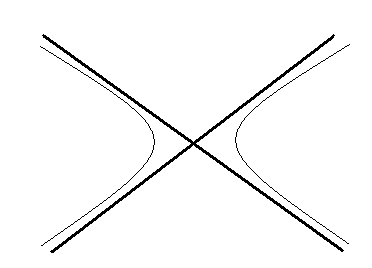The Hyperbola - Tutorial, Problems, Solutions, MCQ Quiz/Worksheet - Introducing the Equation, Eccentricity, Axis, Chords, Tangents, Normals

Target Audience: High School Students, College Freshmen and Sophomores, Class 11/12 Students in India preparing for ISC/CBSE and Entrance Examinations like the IIT-JEE  Main or Advanced/AIEEE, and anyone else who needs this Tutorial as a reference!

After reading this tutorial you might want to check out some of our other Mathematics Quizzes as well.
 Quizzes on ProgressionsMCQ #1: Arithmetic Progression MCQ #2: Geometric ProgressionMCQ #3 : More on Geometric Progressions.MCQ #4 : Harmonic Progressions. MCQ #5: More on Harmonic ProgressionMCQ #6: Mixed ProgressionsComplex NumbersMCQ #1MCQ #2: More on Complex NumbersQuadratic EquationsMCQ Quadratic EquationsQuadratic In-equationsMCQ Quadratic In-equations Coordinate Geometry - Straight LinesMCQ #1: Cartesian Planes, Straight Line BasicsMCQ #2 on Straight LinesMCQ #3 on Straight LinesMCQ #4 on Straight LinesCircles1 MCQ #1 on Circles. 2 MCQ #2 on Circles. 3 MCQ #3 on Circles. Conic Sections- Parabola, Hyperbola, Ellipse1 MCQ- The Basics of Conic Sections2 MCQ on Parabola..3 MCQ on Hyperbola4 MCQ on Ellipses. ProbabilityMCQ #1 on Basic ProbabilityMCQ #2: More Challenging Problems on ProbabilityMCQ #3- Conditional Probability and Bayes Theorem

A Quick Outline of what the tutorial will cover

The Hyperbola - The Equation, Vertices, Transverse and Conjugate Axis, Conjugate Hyperbola, Chords and Latus Rectum

• The equation ax2+2hxy+by2+2gx+2fy+c=0 denotes a hyperbola when abc+2fgh-af2-bg2-ch2≠0 and h2-ab>0.
• The hyperbola is the locus of a point in a plane such that the ratio of distance of that point from a fixed point, focus, to the ratio of the distance of that point from a fixed line, the directrix, is constant and is always greater than one.
• The ratio is called eccentricity, denoted by e.
• Like an ellipse, the hyperbola also has two ‘focus’ and correspondingly two ‘directrix’

Important Features of a Hyperbola

Transverse and Conjugate Axis. The line joining the vertices is called the Transverse Axis. The straight line through the centre perpendicular to transverse axis is called the Conjugate Axis.

• A hyperbola can also be defined as the locus of a point which moves in a plane such that the difference of its distances from two fixed points(foci) is always constant.

Note :  The results are for standard form of hyperbola! To get the results of conjugate hyperbola, interchange x and y

• Many results of hyperbola can be obtained by just changing the sign of b2 term. However, one should practice deriving the results by hand to practice working with hyperbola.

Chord:Equation of chord joining (asecα,btanα) and (asecβ,btanβ) is xacosα-β2+y2sinα-β2=cosα+β2Some Important Points To Remember :

• The tangent drawn at any point bisects the angle between the lines, joining the point to the foci, whereas the normal bisects the supplementary angle between the lines.
• The locus of the point of intersection of two perpendicular tangents to a hyperbola is a circle known as the director circle.
• Equation of the director circle is x2 + y2 = a2 – b2.
• From any point (x1, y1) in general two tangents can be drawn to hyperbola. The equation of the pair of tangents is SS1 = T2  where the equation of the chord of contact is T = 0 and the equation of the chord bisected at the point (x1, y1) is T = S1.

Auxillary Circles, Diameters, Conjugate Diameter, Asymptotes, Rectangular Hyperbola

Conjugate Diameters:   Two diameters are conjugate diameters which bisects chords parallel to each other. Condition turns out to be mm1=b2/a2 The only pair of conjugate diameters that are perpendicular to each other and does not satisfy the above condition is the major and minor axis of ellipse. If two conjugate diameters are equal, then they are called equi-conjugate diameters.

• Asymptotes:
• A line which touches the hyperbola at “infinity” is called asymptote.More About Asymptotes of Hyperbolas; Their Equation(s); Angle Between Asymptotes; Rectangular Hyperbola

• The combined equation of Asymptotes is (x/a+y/b)(x/a–y/b) = 0 or xx2/a2 – y2/b2 = 0 which shows that the equation of the asymptote differs from that of the hyperbola in the constant term only. Also the angle between the asymptotes is 2 tan–1 (b/a).
• The lines x2/a2+y2/b2=0 are also asymptotes to the conjugate hyperbola x2/a2–y2/b2=1.
• Any line drawn parallel to the asymptote of the hyperbola would meet the curve only at one point.
• The equation of the hyperbola and that of its pair of asymptotes differ by a constant. For example, if S = 0 is the equation of the hyperbola, then the combined equation of the asymptotes is given by S + K = 0. The constant K is obtained from the condition that the equation S + K = 0 represents a pair of lines. Finally the equation of the corresponding conjugate hyperbola is S + 2K = 0.
• Rectangular hyperbola. If the asymptotes of a hyperbola are at right angles to each other, it is called a rectangular hyperbola. Condition : b = a. Equation: x2-y2=a2

Here are some of the problems solved in this tutorial :

Q: Find the equation of hyperbola whose focus is (1,2), directrix the line x+y+1, and eccentricity is 3/2.

Q: Find the hyperbola whose conjugate axis is 5 and the distance between foci is 13.

Q: Find the equation of hyperbola whose foci are (8,3) (0,3) and eccentricity is 4/3.

Q: Find the hyperbola whose asymptotes are 2x – y = 3 and 3x + y – 7 = 0 and which passes through the point (1, 1).

Q: Prove that the chords of the hyperbola which touch its conjugate hyperbola are bisected at the point of contact.